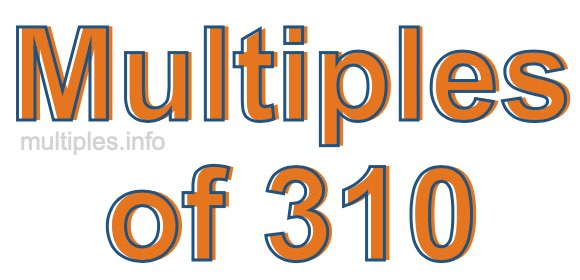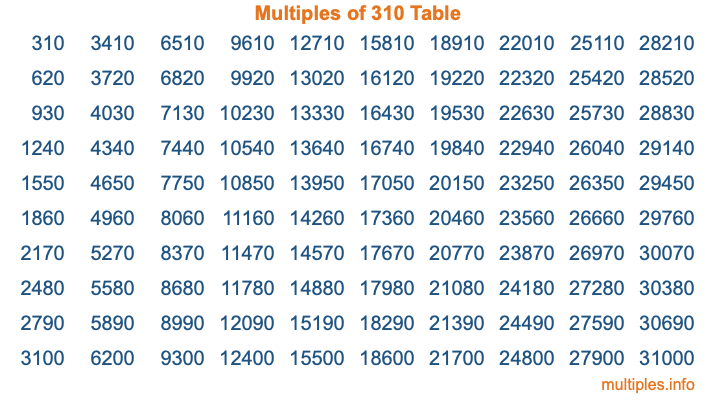Multiples of 310Welcome to the Multiples of 310 page. Here we will first teach you everything you will ever need to know about the multiples of 310, and then give you a study guide summary of everything we taught you to make sure you remember it all. Use this page to look up facts and learn information about the multiples of 310. This page will make you a multiples of three hundred ten expert!

Definition of Multiples of 310
Multiples of 310 are all the numbers that when divided by 310 equal an integer. Each of the multiples of 310 are called a multiple. A multiple of 310 is created by multiplying 310 by an integer.

Therefore, to create a list of multiples of 310, you start with 1 multiplied by 310, then 2 multiplied by 310, then 3 multiplied by 310, and so on for as long as you want. Thus, the list of the first five multiples of 310 is 310, 620, 930, 1240, and 1550. To see a larger list of multiples of 310, see the printable image of Multiples of 310 further down on this page. We also have a category where you can choose any nth multiple of 310.

Multiples of 310 Checker
The Multiples of 310 Checker below checks to see if any number of your choice is a multiple of 310. In other words, it checks to see if there is any number (integer) that when multiplied by 310 will equal your number. To do that, we divide your number by 310. If the the quotient is an integer, then your number is a multiple of 310.

Is  a multiple of 310?

Least Common Multiple of 310 and ...
A Least Common Multiple (LCM) is the lowest multiple that two or more numbers have in common. This is also called the smallest common multiple or lowest common multiple and is useful to know when you are adding our subtracting fractions. Enter one or more numbers below (310 is already entered) to find the LCM.

Check out our LCM Calculator if you need more details about the Least Common Multiple or if you need the LCM for different numbers for adding and subtraction fractions.

nth Multiple of 310
As we stated above, 310 is the first multiple of 310, 620 is the second multiple of 310, 930 is the third multiple of 310, and so on. Enter a number below to find the nth multiple of 310.

th multiple of 310

Multiples of 310 vs Factors of 310
310 is a multiple of 310 and a factor of 310, but that is where the similarities end. All postive multiples of 310 are 310 or greater than 310. All positive factors of 310 are 310 or less than 310.

Below is the beginning list of multiples of 310 and the factors of 310 so you can compare:

Multiples of 310: 310, 620, 930, 1240, 1550, etc.

Factors of 310: 1, 2, 5, 10, 31, 62, 155, 310

As you can see, the multiples of 310 are all the numbers that you can divide by 310 to get a whole number. The factors of 310, on the other hand, are all the whole numbers that you can multiply by another whole number to get 310.

It's also interesting to note that if a number (x) is a factor of 310, then 310 will also be a multiple of that number (x).

Multiples of 310 vs Divisors of 310
The divisors of 310 are all the integers that 310 can be divided by evenly. Below is a list of the divisors of 310.

Divisors of 310: 1, 2, 5, 10, 31, 62, 155, 310

The interesting thing to note here is that if you take any multiple of 310 and divide it by a divisor of 310, you will see that the quotient is an integer.

Multiples of 310 Table
Below is an image of the first 100 multiples of 310 in a table. The table is in chronological order, column by column. The first column has the first ten multiples of 310, the second column has the next ten multiples of 310, and so on.The Multiples of 310 Table is also referred to as the 310 Times Table or Times Table of 310. You are welcome to print out our table for your studies.

Negative Multiples of 310
Although not often discussed or needed in math, it is worth mentioning that you can make a list of negative multiples of 310 by multiplying 310 by -1, then by -2, then by -3, and so on, to get the following list of negative multiples of 310:

-310, -620, -930, -1240, -1550, etc.

Multiples of 310 Summary
Below is a summary of important Multiples of 310 facts that we have discussed on this page. To retain the knowledge on this page, we recommend that you read through the summary and explain to yourself or a study partner why they hold true.

There are an infinite number of multiples of 310.

A multiple of 310 divided by 310 will equal a whole number.

310 divided by a factor of 310 equals a divisor of 310.

The nth multiple of 310 is n times 310.

The largest factor of 310 is equal to the first positive multiple of 310.

310 is a multiple of every factor of 310.

310 is a multiple of 310.

A multiple of 310 divided by a divisor of 310 equals an integer.

310 divided by a divisor of 310 equals a factor of 310.

Any integer times 310 will equal a multiple of 310.

Multiples of a Number
Here you can get the multiples of another number, all with the same attention to detail as we did for multiples of 310 on this page.

Multiples of
Multiples of 311
Did you find our page about multiples of three hundred ten educational? Do you want more knowledge? Check out the multiples of the next number on our list!